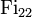# Fischer group:Fi22

Jump to: navigation, search
This article is about a particular group, i.e., a group unique upto isomorphism. View specific information (such as linear representation theory, subgroup structure) about this group
View a complete list of particular groups (this is a very huge list!)[SHOW MORE]

## Definition

This group, denoted$\operatorname{Fi}_{22}$, is one of the three Fischer groups. Fischer groups are 3-transposition groups that are not the symmetric groups or alternating group.$\operatorname{Fi}_{22}$ is one of the 26 sporadic simple groups that appear in the classification of finite simple groups.

The order of the group is:$64561751654400 = 2^{17} \cdot 3^9 \cdot 5^2 \cdot 7 \cdot 11 \cdot 13$

## Arithmetic functions

Function Value Similar groups Explanation for function value
order (number of elements, equivalently, cardinality or size of underlying set) 64561751654400 groups with same order
number of conjugacy classes 65